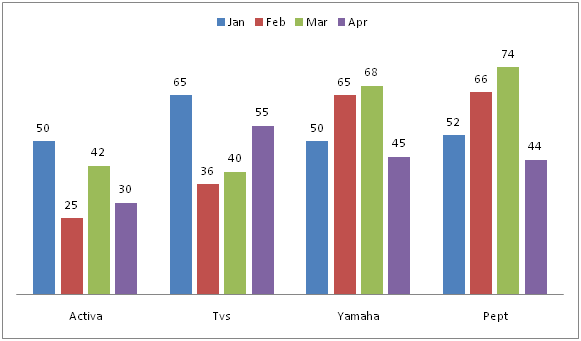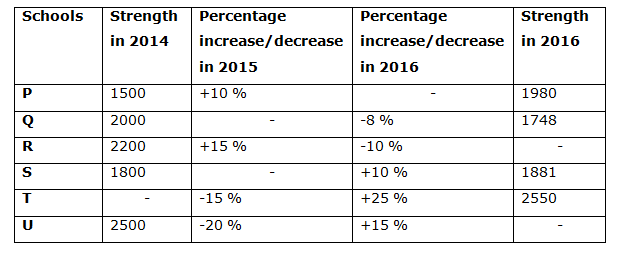# Quantitative Aptitude Questions (Data Interpretation) for RRB Assistant Mains 2018 Day-175

Dear Readers, IBPS RRB is conducting Online Examination for the recruitment of Assistant. To enrich your preparation here we have providing new series of Data Interpretation – Quantitative Aptitude Questions. Candidates those who are appearing in RRB Assistant Mains Exams can practice these Quantitative Aptitude average questions daily and make your preparation effective.

[WpProQuiz 3341]

Click “Start Quiz” to attend these Questions and view Solutions

Directions (1 – 5): Study the following information carefully and answer the given questions.

Following bar graph shows the monthly sales turn over (In lakhs) of various scooters in 5 different months.1) Total sales turnover of Activa in all the given months together is approximately what percentage of total sales turnover of all the given scooters in the month of Feb?

a) 60 %

b) 70 %

c) 77 %

d) 95 %

e) 115 %

2) Find the average sales turnover of Yamaha in all the given months together?

a) 57 lakhs

b) 63 lakhs

c) 52 lakhs

d) 68 lakhs

e) None of these

3) Find the difference between the total sales turnovers of all the given scooters in the month of Jan to that of Apr?

a) 36 lakhs

b) 32 lakhs

c) 25 lakhs

d) 43 lakhs

e) None of these

4) Find the respective ratio between the total sales turnovers of Pept in all the given months together to that of total sales turnover of all the given scooters in the month of mar?

a) 34 : 31

b) 59 : 56

c) 42 : 37

d) 12 : 5

e) None of these

5) Total sales turnover of Activa in Mar and Apr together is approximately what percentage more/less than the total sales turnover of Tvs in Feb and Mar together?

a) 15 % less

b) 10 % less

c) 5 % less

d) 5 % more

e) 10 % more

Total sales turnover of Activa in Mar and Apr together

= > 42 + 30 = 72 lakhs

Total sales turnover of Tvs in Feb and Mar together

= > 36 + 40 = 76 lakhs

Required % = [(76 – 72)/76]*100 = 5 % less

Directions (6 – 10): Study the following information carefully and answer the given questions.

The following table shows the strength of six different schools in 2014 and 2016 and also shows the percentage increase/decrease in 2015 and 2016. Some data are missing.NOTE:

6) Find the sum of strength of school U in 2015 and the strength of school R in 2016?

a) 3456

b) 2389

c) 4277

d) 4215

e) None of these

7) Percentage increase/decrease in the strength of school P in 2016 is what percent of the percentage increase/decrease in the strength of school Q in 2015?

a) 450 %

b) 400 %

c) 320 %

d) 250 %

e) None of these

8) Strength of school T in 2014 is what percentage more than the strength of school Q in 2014?

a) 20 %

b) 18 %

c) 15 %

d) 22 %

e) None of these

9) Find the sum of strength of all the schools in 2016?

a) 13245

b) 12736

c) 11637

d) 14235

e) None of these

10) Find the respective ratio of the percentage increase/decrease in the strength of S in 2015 and that of P in 2016?

a) 9 : 4

b) 2 : 3

c) 1 : 4

d) 3 : 2

e) None of these

Total sales turnover of Activa in all the given months together

= > 50 + 25 + 42 + 30 = 147 lakhs

Total sales turnover of all the given scooters in the month of Feb

= > 25 + 36 + 65 + 66 = 192 lakhs

Required % = (147/192)*100 = 76.56 % = 77 %

The average sales turnover of Yamaha in all the given months together

= > (50 + 65 + 68 + 45)/4

= > 228/4 = 57 lakhs

The total sales turnover of all the given scooters in the month of Jan

= > 50 + 65 + 50 + 52 = 217 lakhs

The total sales turnover of all the given scooters in the month of Apr

= > 30 + 55 + 45 + 44 = 174 lakhs

Required difference = 217 – 174 = 43 lakhs

The total sales turnover of Pept in all the given months together

= > 52 + 66 + 74 + 44 = 236

The total sales turnover of all the given scooters in the month of mar

= > 42 + 40 + 68 + 74 = 224

Required ratio = 236: 224 = 59: 56

Total sales turnover of Activa in Mar and Apr together

= > 42 + 30 = 72 lakhs

Total sales turnover of Tvs in Feb and Mar together

= > 36 + 40 = 76 lakhs

Required % = [(76 – 72)/76]*100 = 5 % less

Strength of school U in 2015 = 2500*(80/100) = 2000

Strength of school R in 2016 = 2200*(115/100)*(90/100) = 2277

Required sum = 2000 + 2277 = 4277

Let percentage increase/decrease in the strength of school P in 2016 = x%

And percentage increase/decrease in the strength of school Q in 2015 = y%

Now,

1500*(110/100)*[(100 + x)/100] = 1980

= > (100 + x)/100 = 1980*(100/110)*(1/1500)

= > (100 + x)/100 = 6/5

= > 100 + x = 120

= > x = 20 => 20% increase

And

2000*[(100 + y)/100]*(92/100) = 1748

= > (100 + y)/100 = 1748*(100/92)*(1/2000)

= > (100 + y)/100 = 437/460

= > 100 + y = 95

= > y = -5 = > 5% decrease

Required % = (20/5)*100 = 400%

Let the strength of school T in 2014 be y,

Y*(85/100)*(125/100) = 2550

=> y = 2550*(100/125)*(100/85)

=> y = 2400

Required % = {(2400 – 2000)/2000}*100 = (400/2000)*100 = 20 %

Strength of school R in 2016 = 2200*(115/100)*(90/100) = 2277

Strength of school U in 2016 = 2500*(80/100)*(115/100) = 2300

Required sum = 1980 + 1748 + 2277 + 1881 + 2550 + 2300 = 12736

Let percentage increase/decrease in the strength of school S in 2015 = x%

And percentage increase/decrease in the strength of school P in 2016 = y%

1800*[(100 + x)/100]*(110/100) = 1881

=> (100 + x)/100 = 1881*(100/110)*(1/1800)

=> (100 + x)/100 = 19/20

=> 100 + x = 95

=> x = -5 => 5 % decrease

1500*(110/100)*[(100 + y)/100] = 1980

=> (100 + y)/100 = 1980*(100/110)*(1/1500)

=> (100 + y)/100 = 6/5

=> 100 + y = 120

=> y = 20 => 20 % increase

Required ratio = 5: 20 = 1: 4

Daily Practice Test Schedule | Good Luck

 Topic Daily Publishing Time Daily News Papers & Editorials 8.00 AM Current Affairs Quiz 9.00 AM Quantitative Aptitude “20-20” 11.00 AM Vocabulary (Based on The Hindu) 12.00 PM General Awareness “20-20” 1.00 PM English Language “20-20” 2.00 PM Reasoning Puzzles & Seating 4.00 PM Daily Current Affairs Updates 5.00 PM Data Interpretation / Application Sums (Topic Wise) 6.00 PM Reasoning Ability “20-20” 7.00 PM English Language (New Pattern Questions) 8.00 PM